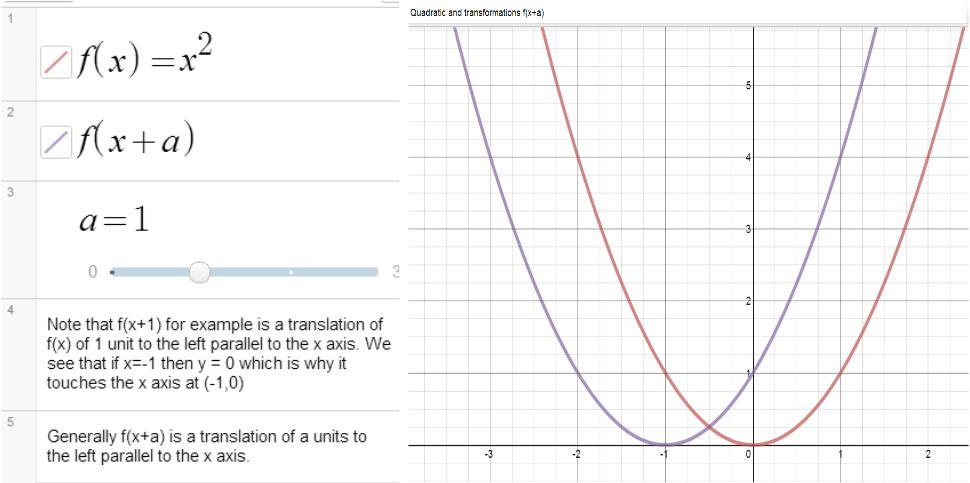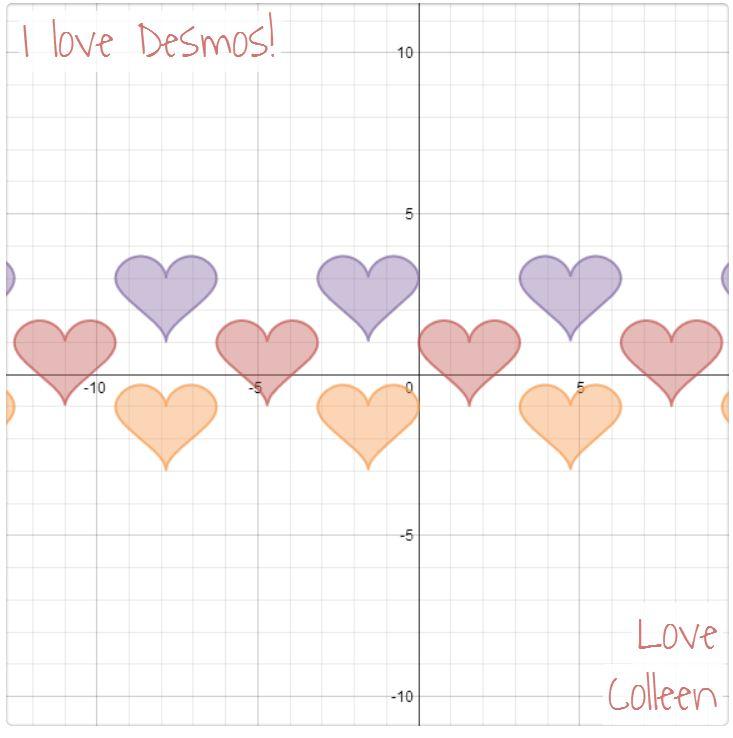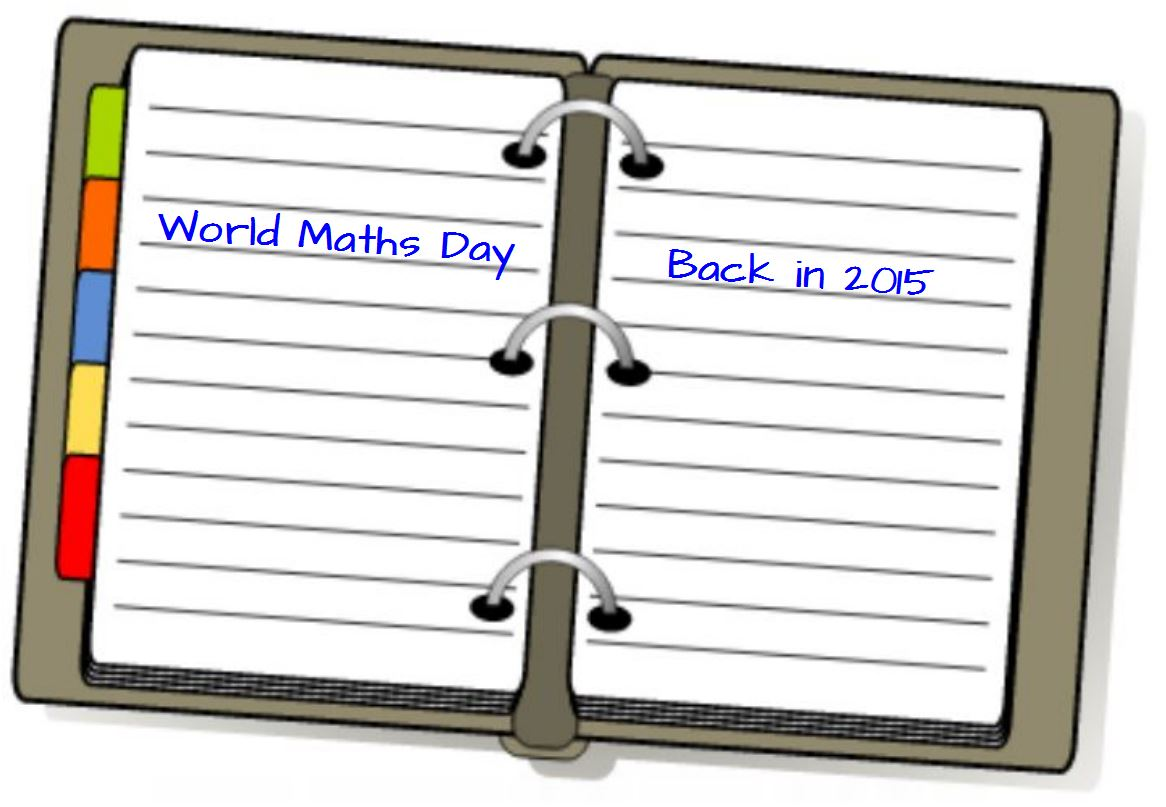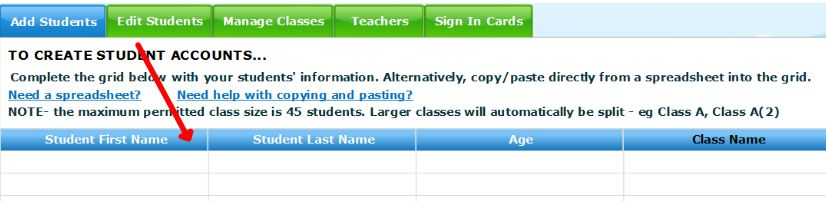# Graphs and TransformationsNote how easy it is using the Desmos graphing calculator to show a graph and then the same graph after a transformation. For example see here the graph of x2 and (x+a)(or click on  the image).

As you can see all you need do is define f(x) which then gives you the ability to graph transformations of f(x). Using a slider means students can explore what happens if the value of a changes. In this particular example a is restricted to integer values from 0 to 3 but this is very easy to change by editing the slider; simply click on the numbers at the ends of the slider and choose the values required, you can also choose the step size.

I like the ability to add text to graph pages which means it is possible to add a few words of explanation or even questions for students.

I have used these prompts before in a computer room, the aim being for students to explore and generalize. The headings are links to Desmos graph pages students could experiment with.

Transformations Exercise

For linear, quadratic and the sine and cosine functions the following transformations should be understood:

y=f(x)+a trigonometric
Eg plot y = x2   and y = x+ 3   on the same diagram, compare the graphs.
Plot y = x− 4, y = x− 1
Compare y = x2  and y = x+ k where k is any integer, positive or negative.
Also compare y = sin x and y = sin x + 3.
Compare y = sin x and y = sin x + k where k is any number

y = f(x+a) trigonometric
Eg plot  y = x2   and y = (x+ 3)on the same diagram, compare the graphs.
Compare y = x2   and y = (x−3)2.  Compare y = x2   and y = (x−4)2
Compare y = x2  and y =(x+ k)2 where k is any integer, positive or negative.
Compare y = sin x and y = sin (x+90) , sin (x – 90), sin(x+180)
Compare y = cos x with y = cos(x+90), cos (x-90)

3. y = af(x) trigonometric
Compare y = sinx and y = 2sinx.
Compare y = cosx and y = 3cosx and y =  0.5cos x
Compare y=x2 and kx2 where k is any integer positive or negative.

4. y = f(ax) trigonometric
Compare y = sinx and y = sin2x. Compare y = sin x and y = sin(0.5x).
Compare y = cos x and y = cos 3x. Compare y = cos x and y = cos(0.5x)

Update – I must add these reactions from Twitter after publishing this post, from @Desmos (click on the image for a great example on transformations of points).and this fabulous Umbrella and Rain from Luke Walsh, try the slider and watch the rain and umbrella!# Send a math-o-gram …

…to the one you love – or any of your mathematical friends!

Note that this was a special for Valentine’s Day – look out for this next year!Choose one of the supplied romantically mathematical designs, add a custom message and send it off! Being a Desmos math-o-gram there’s more – using the slider, the hearts on this design will dance! (Click on the image).Sending Valentine good wishes to mathematicians everywhere!

….and in case you have not used Desmos before you might find some of the posts on this page useful.

By Colleen Young Posted in Desmos

# Here’s the question….

I have previously mentioned Zeb Friedman’s Grade that Question resources on the Suffolk Maths site; this such a good idea and will certainly help students become familiar with what is required at each level or grade. (See GCSE revision materials, Foundation Tier Materials and Higher Tier Materials).

There are many other resources where questions are given by level or grade, also resources to help students understand the various levels / grades; I have included useful links on the Progression page.

It struck me that grading the questions would make an ideal starter, this could then seamlessly turn into a main activity where students try the questions! I have therefore added this to the Starters site, I have also added some posts from this blog so anything I think useful for a starter is in one place. Note Here’s the Diagram ….what’s the question? (with additions since I first wrote the post), Settlers and Ever wondered why; Year 11 will be getting the fractional and negative indices ‘Ever wondered why’ worksheet from Just Maths as a starter this week!

It struck me whilst writing this post that we could turn the title round, supply the students with a topic and ask for a grade …. question. We could of course also turn round Here’s the Diagram ….what’s the question – let them read a wordy description and then draw the diagram – perhaps a useful exercise for Mechanics A Level students.

Now wondering about other possibilities for Here’s the ….. , what’s the …. ? resources!

# World Maths DayThe World Education Games 2013 was held on 5th to 7th March 2013 with World Maths Day on 6th March 2013.

The World Maths Day blog has the latest news you can also follow @WorldEduGames on Twitter or The World Education Games on Facebook.

During the World Maths Day event students of all ages play other students in their age category from around the world in live games of mental arithmetic. I have seen many students of all ages thoroughly enjoying this event.

During the event itself students can earn points for up to 50 games, 10 at each level, each game lasting one minute. Over 50 games can be played but the points will not be added to their personal score for the event.  Teachers can play too! Note that the event lasts as long as it is the event date somewhere in the world; check the site for start times at various cities round the world; in London for example the event lasts from 10am on 5th March to 10am on 7th March. There are also clear guides for students and teachers available under Resources.

For those interested in practising their spelling in preparation for World Literacy Day there are numerous resources online including games.

Have fun!Current high score on level 2 (19+ age category)!

# World Maths Day Registration

Having been asked by more than one person last year how I added my students I thought I’d post some instructions.

When I first logged into the site I found I was on the Add Students page where you will find instructions. I had already created a spreadsheet with the required information, first name, last name, age and class name. Copy your spreadsheet by selecting all the cells then use Ctrl – c. Now go back to the site and click in the first cell in the header columns it may look at this point that this is impossible!  But click in it anyway then paste – Ctrl-vHopefully your student or students should appear in the grid – scroll down and select ‘Submit’. The first time I tried I received an error message that some surnames were too long, so I shortened them and tried again – success, the whole school – over 1000 students enrolled. Teachers can play too – I love the age category: 19+!